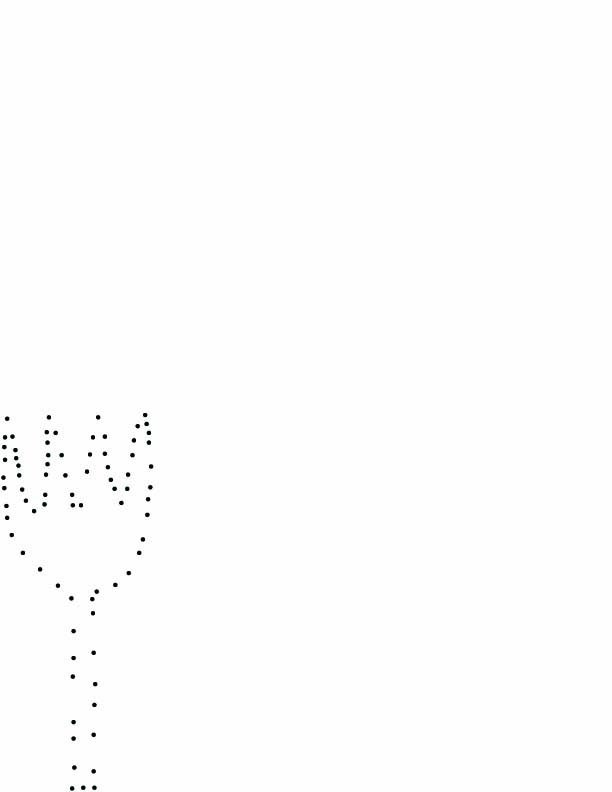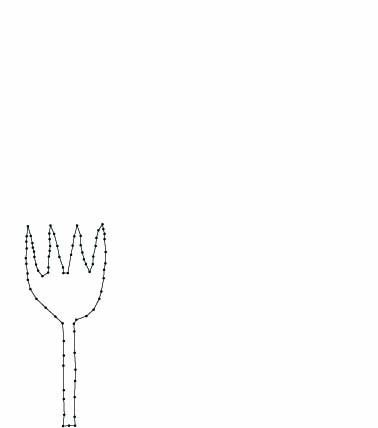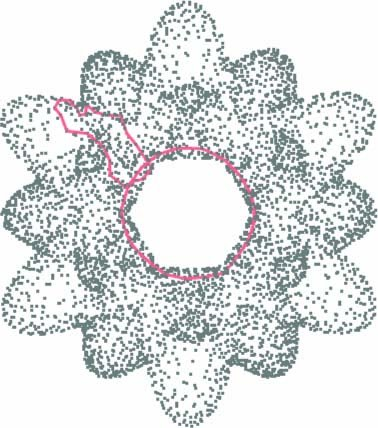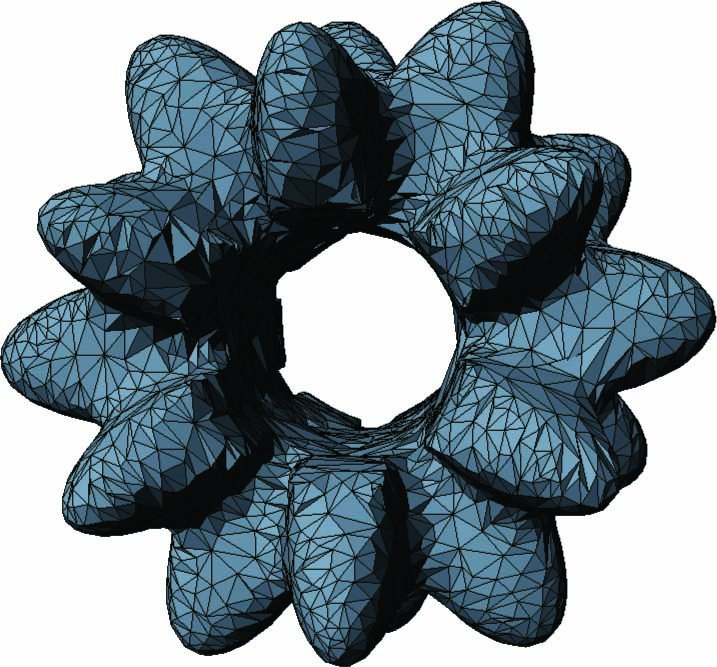# Institut für Theoretische Informatik

## Vortragszusammenfassung

Curve and Surface Reconstruction

Curve and surface reconstruction from a set of sample points is a problem occurring in many domains, e.g., reverse engineering, CAD, and data interpretation. Given a finite set S of sample points from an unknown curve or surface γ, reconstruct the curve or surface. In the case of a curve this means to connect two sample points if and only if they are adjacent on γ and in the case of a surface this means to construct a triangulated surface with vertices in S homeomorphic to γ. The figures below show sample sets and the reconstructed curve or surface, respectively.The problems are well-studied since the early 80s and a number of effective heuristics were developed in the 80s and 90s. In the past 10 years, the research shifted to algorithms which come with a guarantee, i.e., which provably solve the problem for a certain class of curves or surfaces and for samples satisfying a certain sampling condition.

The first algorithms worked for smooth closed curves. This was then extended to collections of open and closed smoothed curves, and finally to non-smooth curves (the example on the left illustrates such a reconstruction). In the case of surfaces, the case of smooth surfaces is solved. Current work investigates generalizations to non-smooth surfaces.